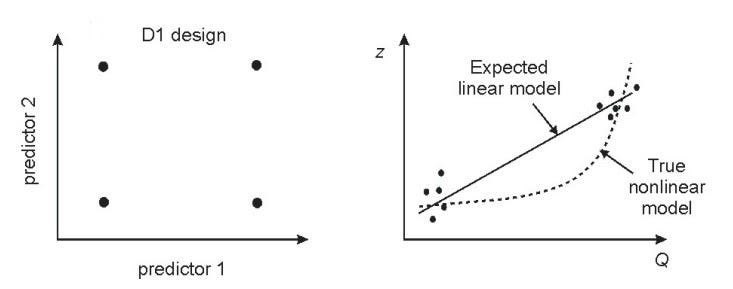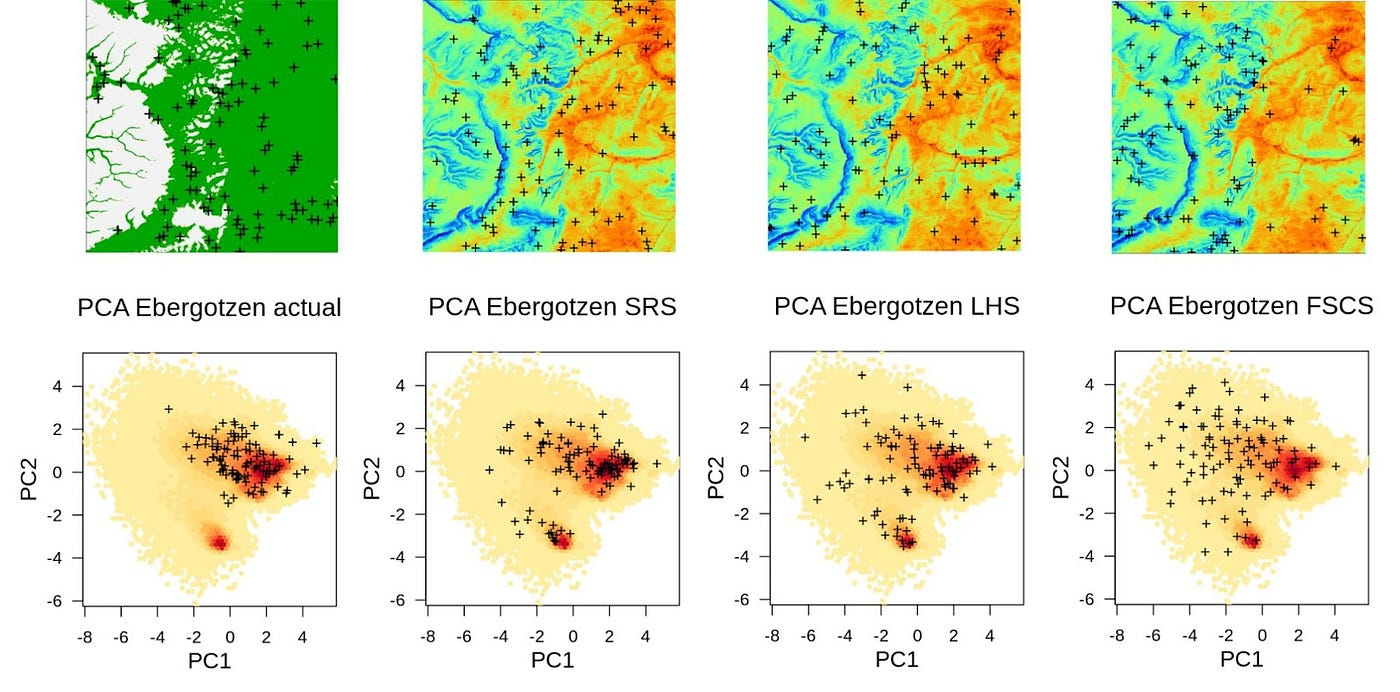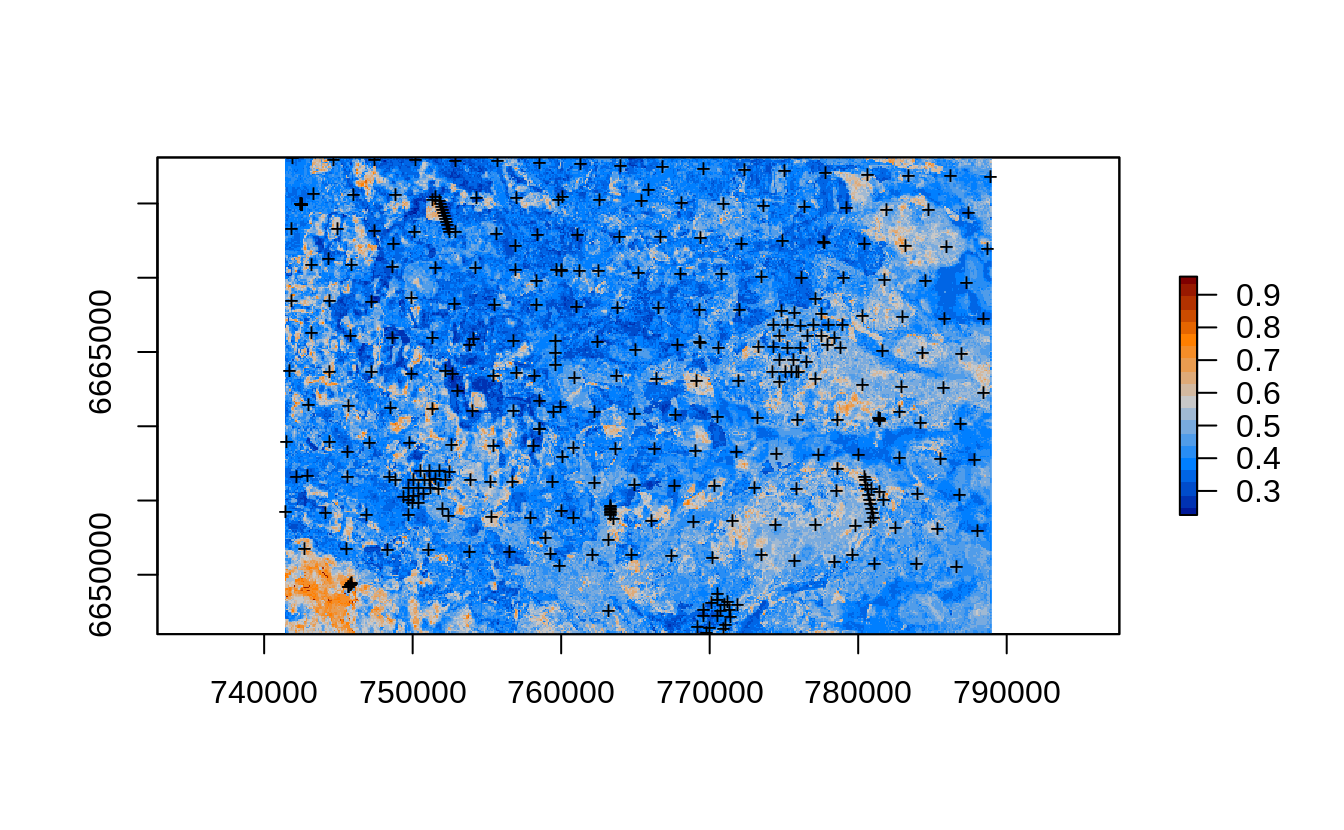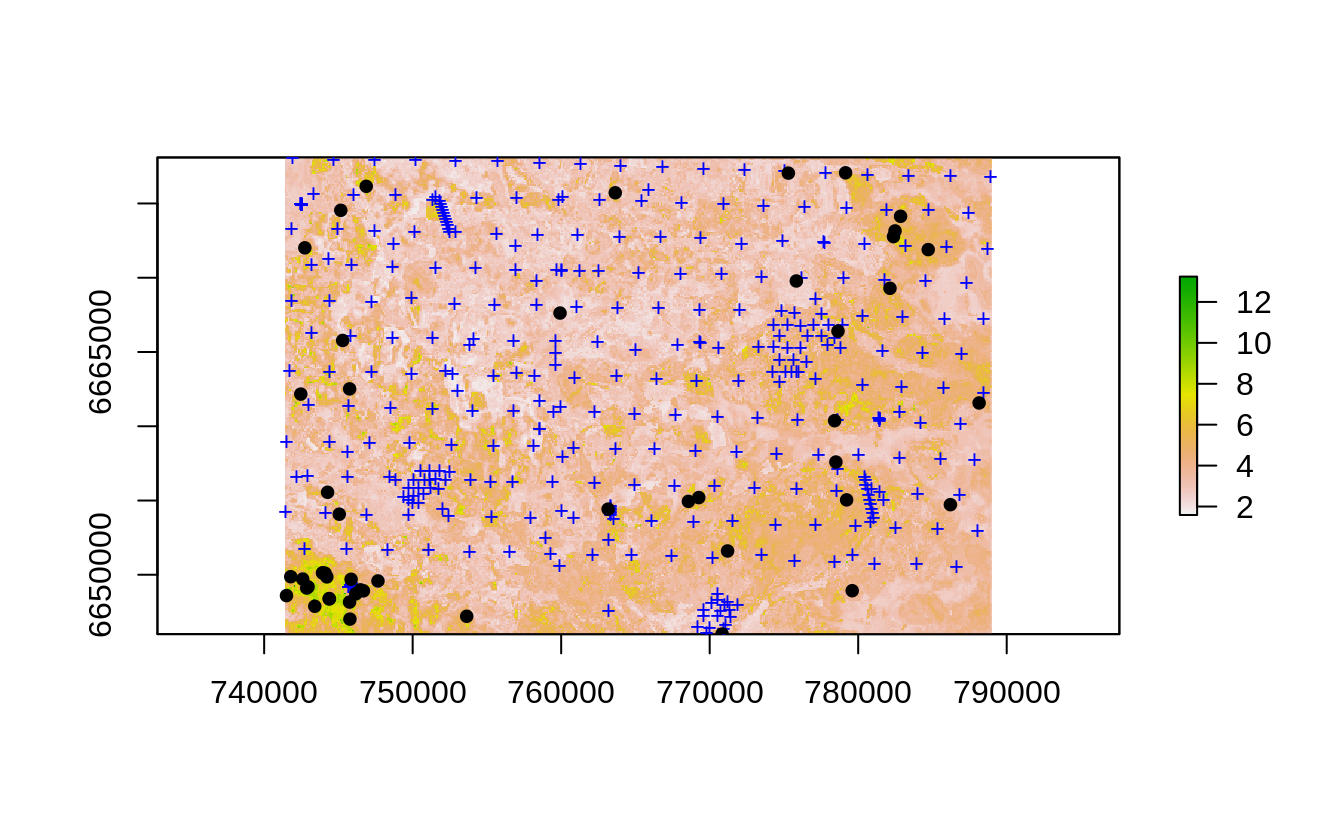# Spatial sampling and resampling and Machine Learning: A tutorial in R

Prepared by: Tom Hengl (OpenGeoHub), Leandro Parente (OpenGeoHub) and Ichsani Wheeler (OpenGeoHub)

Samples collected in soil, vegetation science, ecology, geology can be used to build predictive mapping models and produce predictions i.e. maps. Increasingly, Machine Learning methods such Random Forest and similar are used to train models and produce maps and estimate population parameters. But how to design sampling plans for Machine Learning models and should you worry if your sampling is based on subjectively allocated points? Should you worry about extrapolation and spatial clustering of points? How to ensure that the ML models fitted using given point data are representative / unbiased?

# Spatial Sampling

Sampling in statistics is done for the purpose of estimating population parameters and/or for testing of experiments. If Observations and Measurements (O&M) are collected in space and using e.g. GPS this is referred to as spatial sampling and is often materialized as a point map with points representing locations of planned or implemented O&M. Preparing a spatial sampling plan is a type of design of experiment and hence it is important to do it right to avoid any potential bias.

Spatial sampling or producing and implementing sampling designs are common in various fields including physical geography, soil science, geology, vegetation science, ecology and similar. Imagine an area that potentially has problems with soil pollution by heavy metals. If we collect enough samples, we can overlay points vs covariate layers, then train spatial interpolation / spatial prediction models and produce predictions, map, of the target variable. For example, to map soil pollution by heavy metals or soil organic carbon stock, we can collect soil samples on e.g. a few hundred predefined locations, then take the samples to the lab, measure individual values and then interpolate them to produce a map of concentrations. This is one of the most common methods of interest of geostatistics where e.g. various kriging methods are used to produce predictions of the target variable (see e.g. Bivand et al., 2014).

There are many sampling design algorithms that can be used to generate spatial sampling locations. In principle, all spatial sampling approaches can be grouped based on the following four aspects:

1. How objective is it? Here two groups exist: (1) objective sampling designs which are either probability sampling or some experimental designs from spatial statistics; (2) subjective or convenience sampling which means that the inclusion probabilities are unknown and are often based on convenience e.g. distance to roads / accessibility;
2. How much identically distributed is it? Here at least three groups exist: (1) Independent Identically Distributed (IID) sampling designs, (2) Clustered sampling i.e. non-equal probability sampling, and (3) Censored sampling,
3. Is it based on geographical or feature space? Here at least three groups exist: (1) Geographical sampling i.e. taking into account only geographical dimensions + time; (2) Feature-space sampling i.e. taking into account only distribution of points in feature space; (3) Hybrid sampling i.e. taking both feature and geographical space into account;
4. How optimized is it? Here at least two groups exist: (1) Optimized sampling so that the target optimization criteria reaches minimum / maximum i.e. it can be proven as being optimized, (2) “Unoptimized” sampling, when either optimization criteria can not be tested or is unknown,

Doing sampling using objective sampling designs is important as it allows us to test hypotheses and produce unbiased estimation of population parameters or similar. Many spatial statisticians argue that only previously prepared, strictly followed randomized probability sampling can be used to provide an unbiased estimate of the accuracy of the spatial predictions (Brus, 2021). In the case of probability sampling, calculation of population parameters is derived mathematically i.e. estimation process is unbiased and independent of the spatial properties of the target variable (e.g. spatial dependence structure and/or statistical distribution). For example, if we generate sampling locations using Simple Random Sampling (SRS), this sampling design has the following properties:

1. It is an IID with each spatial location with exactly the same inclusion probability,
2. It is symmetrical in geographical space meaning that about the same number of points can be found in each quadrant of the study area,
3. It can be used to derive population parameters (e.g. mean) and these measures are per definition unbiased,
4. Any random subset of the SRS is also a SRS,

SRS is in principle both objective sampling and IID sampling and can be easily generated provided some polygon map representing the study area. Two other somewhat more complex sampling algorithms with similar properties as SRS are for example different versions of tessellated sampling. The generalized random tessellation stratified (GRTS) design was for example used in the USA to produce sampling locations for the purpose of geochemical mapping (Smith et al., 2013); a multi-stage stratified random sampling design was used to produce LUCAS soil monitoring network of points (Tóth et al., 2014).

# Spatial sampling for regression modeling

Large point datasets representing observations and/or measurements in-situ can be used to generate maps by fitting regression and classification models using e.g. Machine Learning algorithms, then applying those models to predict values at all pixels. This is referred to as Predictive Mapping. In reality, many point datasets we use in Machine Learning for predictive mapping do not have ideal properties i.e. are neither IID nor are probabilities of inclusion known. Many are in fact purposive, convenience sampling and hence potentially over-represent some geographic features, are potentially censored and can lead to significant bias in estimation.

If the objective of modeling is to build regression models (correlating the target variable with a number of spatial layers representing e.g. soil forming factors), then we are looking at the problem in statistics known as the response-surface experimental designs. Consider the following case of one target variable (Y) and one covariate variable (X). Assuming that the two are correlated linearly (i.e. Y = b0 + b1 * X), one can easily prove that the optimal experimental design is to (a) determine min and max of X, then put half of the point at Xmin and the other half at Xmax (Hengl et al., 2004). This design is called the D1 optimal design and indeed it looks relatively simple to implement. The problem is that it is the optimal design ONLY if the relationship between Y and X is perfectly linear. If the relationship is maybe close to quadratic than the D1 design is much worse than for example the D2 design (half of points in the middle, half on the edges of feature space).Example of D1 design: (left) D1 design in 2D feature space, (right) D1 design is optimal only for linear model, if the model is curvilinear, it is in fact the worse design than simple random sampling. Image source: Hengl et al. (2004).

In practice, we may not know what is the nature of the relationship between Y and X, i.e. we do not wish to take a risk and produce biased estimation. Hence we can assume that it could be a curvilinear relationship and so we need to sample uniformly in the feature space. One such sampling design well known in statistics is the Latin Hypercube sampling. In a nutshell, LHS sampling is based on dividing Cumulative Density Function (CDF) into n equal partitions, and then choosing a random data point in each partition. The “hypercube” indicates that there are many covariate variables (X) forming a hypercube.

From that point of view of ML, what is especially interesting for Predictive Mapping is to use sampling designs that are based on optimization of sampling in feature space. In the further text we will hence focus on the following four spatial sampling algorithms:

1. Subjective or convenience sampling (here mentioned only for illustration),
2. Simple Random Sampling (SRS),
3. Latin Hypercube Sampling (LHS),
4. Feature Space Coverage Sampling (FSCS),

In principle, SRS and LHS are both Independent Identically Distributed (IID) sampling designs which means that all items in the sample are taken from the same probability distribution + they are all independent (meaning: all sample items are independent events not connected to each other in any way). FSCS is more complex as points are selected based on clustering in feature space and assuming that study area has highly diverse areas (both high and low terrain diversity) it is difficult to predict how the final FSCS samples would look like. For a complete overview of spatial sampling techniques please refer to Brus (2021).

Figure below shows differences between the above mentioned sampling algorithms in both geographical and feature spaces. In this case: actual sampling is significantly missing the whole cluster in feature space, while FSCS seems to show the highest spread in the feature space and by many authors is recognized as the most advantageous sampling design for predictive mapping (Ma et al., 2020). Such sampling diagnostics / comparisons geographical vs feature space help us detect any possible problems before we start running ML.Comparing sampling designs: convenience sampling (actual), Simple Random Sample (SRS), Latin Hypercube Sampling (LHS), and Feature Space Coverage Sampling (FSCS). Points shown in geographical (above) and feature space (below; with first 2 principal components as x, y coordinates).

# Does ML require probability sampling to generate usable maps?

In principle, Machine Learning can generate models and predictions from any training data: technically speaking not a problem. However, any bias in sampling might propagate to any conclusions we make from analysis. Eventually, producing biased or over-optimistic estimates can degrade the user’s confidence in your spatial analysis. Fortunately, there are plenty of diagnostics tools in R (e.g. thanks to the spatstat, and maxlike packages) to help you with checking how representative the training points are, where are the potential extrapolation areas? and how much could sampling design affect estimation of the model parameters? In the Rmarkdown tutorial we specifically guide you through real-data set data science problems and demonstrate how to navigate through such problems. In principle, we suggest three main strategies to reduce impact of sampling bias on predictive mapping:

1. Sub-setting unknown sampling designs so they match as much as possible some probability sampling design,
2. Testing accuracy performance of Machine Learning using data resampling with blocking,
3. Using Ensemble Machine Learning with a combination of linear (simple) and non-linear models to avoid over-fitting and extrapolation blunders,

These steps are explained in detail in this tutorial. Another useful thing to do to estimate any extrapolation problems is to produce: (a) map of prediction errors, and/or (b) map of Area of Applicability of the fitted ML model (Meyer & Pebesma, 2021). Map of prediction errors shows where the predictions are especially poor and can help limit the decisions. The users have a right to know what are the risks connected with the predictions / maps they use.Map or prediction errors (log-soil organic carbon content for topsoil) produced using Ensemble ML. Prediction error maps highlight areas where the models perform poorly; high predictions errors often match the extrapolation areas. Where prediction errors exceed some critical threshold, map is of little use or should not be used for decision-making at all.

# Spatial sampling in a new area: which sampling algorithm to use?

Imagine you are visiting an area for the first time. You basically have little to no knowledge and definitively no initial point data from the area, but you have a diversity of Earth Observation images, DTM-derivatives and similar GIS layers that you would like to use to map distribution of some feature in space. To prepare a sampling plan we recommend using LHS or FSCS sampling. You do not have to spend all the budget on collecting a large amount of points. You could start with a few hundred samples, use e.g. FSCS algorithm, then fit initial ML models and test producing predictions and prediction error maps.

Recommended steps to prepare a sampling plan include:

1. Prepare all covariate layers (rasters) that you plan to use to fit predictive mapping models; import them to R;
2. Convert covariate layers to Principal Components using the landmap::spc function;
3. Cluster the feature space using the h2o.kmeans function; for smaller number of samples use number of clusters equal to number of sampling locations;
4. Generate a sampling design and export the points to GPX format so they can be imported to a hand-held GPS or similar. For fieldwork we recommend using the ViewRanger app which has useful functionality for field work including planning the optimal routes.

If you are collecting more than a few hundred points, then FSCS could become cumbersome and we hence recommend using LHS sampling. This sampling algorithm spreads points symmetrically in the feature space and ensures that the extrapolation (in feature space) is minimized.

# Resampling existing point samples

Assuming that you are working with existing point data sets (i.e. previously conducted surveys with O&M data), then the technique that might help you with further analysis is called “resampling” meaning: sub-setting existing points for the purpose of establishing more balanced representation of geographical or feature space or similar. Resampling is as important as sampling as it helps you prevent from:

• over-fitting i.e. producing models that are biased and/or over-optimistic;
• missing out covariates that are important but possibly shadowed by the covariates over-selected due to overfitting;
• producing poor extrapolation i.e. generating artifacts or blunders in predictions;
• over-/under-estimating mapping accuracy i.e. producing a biased estimate of model performance;

Resamping methods are discussed in detail in Hastie et al. (2009), Kuhn & Johnson (2013) and Roberts et al. (2017). Resampling is also commonly implemented in many statistical and machine learning packages such as the caret or mlr.

The Rmarkdown tutorial explains how to use resampling methods in combination with Ensemble Machine Learning to help solve multiple problems with over-fitting and extrapolation at once.

# Spatial sampling after the initial model: 2nd, and 3rd round sampling

After we have fitted initial models, a logical thing to do to improve predictions would be to collect additional samples and then re-run analysis (so called “re-analysis modeling”) i.e. add new points to the existing points and then refit the models and re-make the predictions. One principle that seems most logical here is to allocate samples proportionally to map uncertainty i.e. proportional to the probability of prediction errors exceeding some required accuracy level. Stumpf et al. (2017) refers to this as the “uncertainty-guided” sampling. An example of 2nd round sampling with only 50 additional points would look something like this:Example of a 2nd round sampling: locations of initial (+) and 2nd round points (dots) produced using the prediction error map from the initial model.

By locating additional sampling points proportionally to where we experience high prediction errors, there is a good chance that the 2nd round sampling will significantly help improve the mapping accuracy after we add the new points and refit the model. In reality we can not predict how much the accuracy will improve — this can be done only by doing extra field work!

# Summary points

We have reviewed some common spatial sampling techniques that are of interest for predictive mapping with Machine Learning. Choosing the correct sampling and resampling method is important to prevent from over-fitting or missing out some important relationships, but most importantly correct sampling and resampling help avoid making bias in predictions (over- or under-estimation). There are now many diagnostic tools that you can use to estimate potential extrapolation areas, fit models with spatial blocking to prevent over-fitting and from producing blunders in the extrapolation space. We believe that Ensemble Machine Learning with spatial blocking is especially suitable for predictive mapping because it combines strict resampling and reduces the over-fitting properties of some ML algorithms.

In addition, we show in the Rmarkdown tutorial how initial Machine Learning models can be used to prepare additional sampling plans to collect points where the predictions are poorest / most problematic. Assuming that such 2nd round 3rd round sampling points will through re-analysis help improve overall accuracy, Machine Learning can be viewed as a continuous iterative mapping process, with each iteration final maps becoming more and more reliable. Of course, if we can also do this by somewhat saving the survey costs, then this is probably the optimal path to producing quality predictions.

Do you have experiences with sampling and modeling using R or are you just starting to use these tools? Contact us and tell us about your experiences and plans.

# Cited references

1. Bivand, R., Pebesma, E., & Rubio, V. (2014). Applied Spatial Data Analysis with R (2nd ed., p. 401). Heidelberg: Springer. https://asdar-book.org/
2. Brus, D. J. (2021). Spatial Sampling with R. (p. 544). London: Taylor & Francis.
3. Hastie, T. J., Tibshirani, R. J., & Friedman, J. J. H. (2009). The Elements of Statistical Learning: Data Mining, Inference, and Prediction. Springer-Verlag New York.
4. Hengl, T., Nussbaum, M., Wright, M. N., Heuvelink, G. B. M., & Gräler, B. (2018). Random forest as a generic framework for predictive modeling of spatial and spatio-temporal variables. PeerJ, 6, e5518. doi:10.7717/peerj.5518
5. Hengl, T., Rossiter, D. G., & Stein, A. (2004). Soil sampling strategies for spatial prediction by correlation with auxiliary maps. Australian Journal of Soil Research, 41(8), 1403–1422. doi:10.1071/SR03005
6. Kuhn, M., & Johnson, K. (2013). Applied predictive modeling (Vol. 810, p. 595). Springer.
7. Ma, T., Brus, D. J., Zhu, A.-X., Zhang, L., & Scholten, T. (2020). Comparison of conditioned Latin hypercube and feature space coverage sampling for predicting soil classes using simulation from soil maps. Geoderma, 370, 114366. doi:10.1016/j.geoderma.2020.114366
8. Meyer, H., & Pebesma, E. (2021). Predicting into unknown space? Estimating the area of applicability of spatial prediction models. Methods in Ecology and Evolution, 12(9), 1620–1633. doi:10.1111/2041–210X.13650
9. Roberts, D. R., Bahn, V., Ciuti, S., Boyce, M. S., Elith, J., Guillera-Arroita, G., … Thuiller, W. (2017). Cross-validation strategies for data with temporal, spatial, hierarchical, or phylogenetic structure. Ecography, 40(8), 913–929. doi:10.1111/ecog.02881
10. Smith, D.B., Cannon, W.F., Woodruff, L.G., Solano, Federico, Kilburn, J.E., and Fey, D.L., (2013). Geochemical and mineralogical data for soils of the conterminous United States: U.S. Geological Survey Data Series 801, 19 p., https://pubs.usgs.gov/ds/801/.
11. Stumpf, F., Schmidt, K., Goebes, P., Behrens, T., Schönbrodt-Stitt, S., Wadoux, A., … Scholten, T. (2017). Uncertainty-guided sampling to improve digital soil maps. Catena, 153, 30–38. doi:10.1016/j.catena.2017.01.033
12. Tóth, G., Jones, A., Montanarella, L. (eds.) 2013. LUCAS Topsoil Survey. Methodology, data and results. JRC Technical Reports. Luxembourg. Publications Office of the European Union, EUR26102 — Scientific and Technical Research series — ISSN 1831–9424 (online); ISBN 978–92–79–32542–7; doi:10.2788/97922

--

--## OpenGeoHub

Not-for-profit research foundation that promotes open geographical and geo-scientific data and develops open source software.